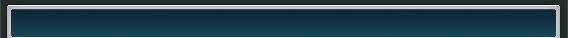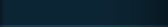Home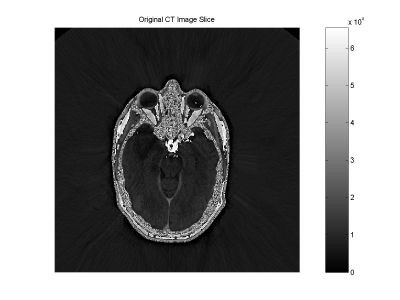Below is an example of the performance improvement using the Constant MTF Resampling Interpolator.  Descriptions of analyses performed on this resampler for non-medical purposes can be found here.

Starting with a slice of a cranial CT scan (512x512, 16 bpp,)
we wish to evaluate the impact of resampling.  To illustrate this, we can interpolate an image 15/32 of a pixel to the left, back 15/32 of a pixel to the right, and subtract the resulting image from the original.

For comparison purposes, we compare this difference using a standard cubic convolution to that using a CMTF convolution.  The first image shows the difference using the cubic, the second image that using the CMTF.  Gray scales are provided to indicate the magnitude of the differences.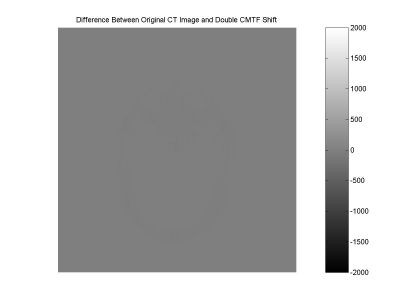It is apparent that the CMTF induces a minimal difference, while the cubic significantly reduces high frequency content in edges and small objects.

Below are zoomed in areas of a small object in the scan.  The first image is from the original scan, the second image is from the original shifted 15/32 of a pixel using the cubic, and the third is from the original shifted 15/32 of a pixel with the CMTF.  The significant blurring by the cubic is obvious.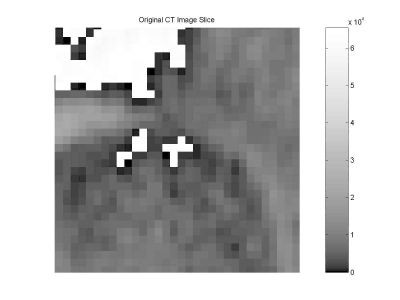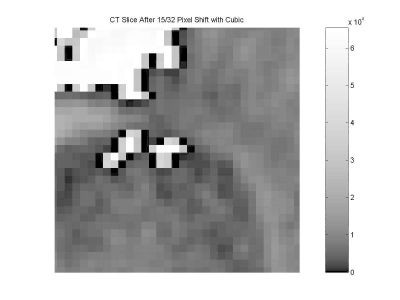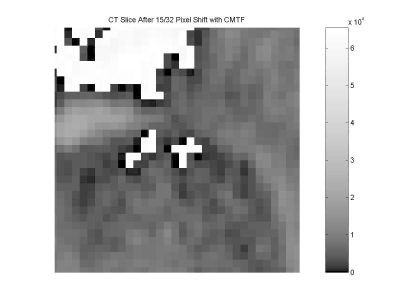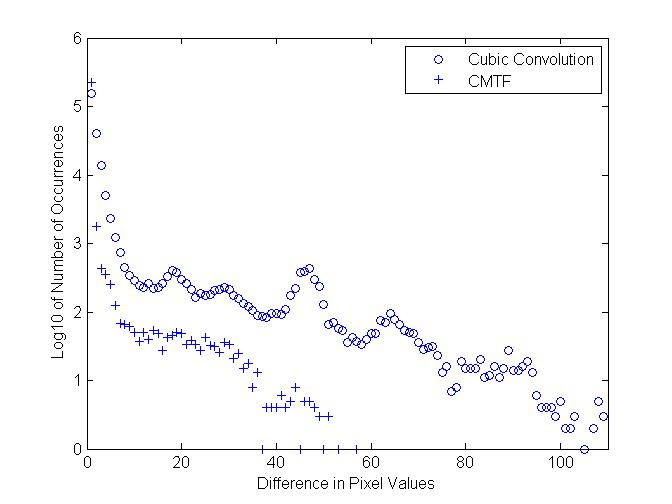The following plot provides the histograms of the difference images between the original CT slice and image interpolated 15/32 of a pixel to the right and then back to the left, for both the CMTF and Cubic Convolution resamplers.  The histograms are plotted using the base 10 logarithm of the number of occurrences to make the whole histogram easier to view.  The histogram of the difference between the original and the cubic convolution image are plotted with circles.  The histogram of the difference between the original and the CMTF image are plotted with plus signs.

Note that while there are differences between the interpolated images and the original, the image interpolated twice with the CMTF is significantly closer to the original than that with the Cubic Convolution.  The residual differences are due to two things:

1) The MTF of the CMTF resampler is not exactly equal to one.  It actually varies from about 0.9986 to 1.0004.  These small variations can occasionally cause a count or two of difference, especially for high pixel values.  Contrast this with the MTF of the Cubic Convolution which can vary from as low as 0.0936 to 1.0.

2) All interpolators suffer from a certain amount of Gibbs Phenomenon, thus causing a small degree of overshoot at sharp edges.  While the CMTF overshoot is very small, especially compared to other interpolators, it can, nevertheless, cause a small difference at very sharp edges in the image.
 Serious Science LLC            Where science fiction becomes fact.